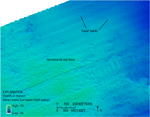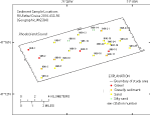U.S. Geological Survey Open-File Report 2011–1005

Surficial Geology of the Sea Floor in Central Rhode Island Sound Southeast of Point Judith, Rhode Island

## SedimentsClick on figures for larger imagesFigure 15. Detailed multibeam bathymetry of trawl marks on the flanks of a hummocky bathymetric high.Figure 17. Location of sediment samples taken during U.S. Geological Survey cruise 2010-033-FA.
##### Sediment Distribution

Sediment samples were taken at 22 of the stations in the study area for grain-size analysis; sea-floor sediment textures at the other 3 stations (996-1, 996-2, and 996-11) were classified based on visual descriptions, as samples could not be obtained due to the coarseness of the sediments. Sediments in the study area range from silty sand located in the bathymetric lows to sand, gravelly sediment, and gravel on the flanks and tops of bathymetric highs (fig. 17). While the floors of the scour depressions and their surrounding areas both tend to be sandy with some areas of gravel, the floors tend to have coarser mean grain sizes than the surrounding areas. The sea floor is composed of sand at 15 of the stations, silty sand at 2, and gravel and gravelly sediment each make up the sea floor at 4 stations. The bathymetric high in the northwestern corner of the study area with the hummocky appearance has gravel and scattered boulders on the sea floor at the two stations in this area (fig. 15). This bathymetric high is probably part of the Point Judith moraine in the 18,000-year-old Harbor Hill-Roanoke Point-Charlestown-Buzzards Bay moraine line that continues to the northwest and south and east of the study area.

##### Sediment Data

The sediment grain-size dataset provided here contains information on the collection, location, description, and texture of sediments at 25 stations occupied during the RV Rafael cruise 2010-033-FA (fig. 17). All analyses were conducted in the Sediment Laboratory at the USGS Woods Hole Coastal and Marine Science Center. Records without textural data and statistics (those with no-data values of -9999) are based on visual descriptions. The basic structure of the data is a flat-file format, a matrix where rows represent individual samples and columns contain sample- and station-specific information. This matrix consists of 42 fields which are defined in the Data Dictionary below.

The sediment data are provided in three formats: a shapefile, Microsoft Excel spreadsheet, and comma-delimited ASCII text file along with metadata in a Zip file located in the GIS Data Catalog section.

##### Data Dictionary

An integral part of any database is the dictionary that explains the structure and content. It contains a list of the fields and definitions of parameters measured. Data utilization is facilitated by reference to this compilation because it defines abbreviations and lists field names.

LABNO - Unique sample identifier assigned in the laboratory

STATIONID - Sample name or number assigned in the field

PROJECT - Project under which samples were taken or data generated

CRUISEID - Name or number of cruise on which sample was collected or station occupied

PRINCIPAL - Name of principal investigator

LATITUDE - Latitude in decimal degrees (north latitudes are positive values)

LONGITUDE - Longitude in decimal degrees (west longitudes are negative values)

DEPTH_M - Depth of water overlying sediment at the time of sampling, measured by a hull-mounted fathometer, corrected for draft, but not corrected for tides, in meters

T_DEPTH - Top depth of the sample below the sediment-water interface, in centimeters

B_DEPTH - Bottom depth of the sample below the sediment-water interface, in centimeters

DEVICE - Device used to collect the sample

MONTH - Number of calendar month during which the sample was collected

DAY - Calendar day on which the sample was collected

YEAR - Calendar year during which the sample was collected

WEIGHT - Dry weight of sample, in grams

ZGRAVEL - Gravel content in percent dry weight of the sample (particles with nominal diameters greater than 2 millimeters; -1 phi and larger)

ZSAND - Sand content in percent dry weight of the sample (particles with nominal diameters less than 2 millimeters but greater than or equal to 0.0625 millimeter; 0 through 4 phi, inclusive)

ZSILT - Silt content in percent dry weight of the sample (particles with nominal diameters less than 0.0625 millimeter but greater than or equal to 0.004 millimeter; 5 through 8 phi, inclusive)

ZCLAY - Clay content in percent dry weight of the sample (particles with nominal diameters less than 0.004 millimeter; 9 phi and smaller)

SEDCLASS - Sediment description based on a rigorous definition (Shepard, 1954)

MEDIAN - Middle point in the grain-size distribution, in phi units

MEAN - Average value in the grain-size distribution, in phi units

STDDEV - Standard deviation (root mean square of the deviations) of the grain-size distribution, in phi units (that is, sorting)

SKEWNESS - Deviation from symmetrical form of the grain-size distribution

KURTOSIS - Degree of curvature near the mode of the grain-size distribution

PHI_11 - Weight percent of the sample in the 11-phi fraction (nominal diameter of particles greater than or equal to 0.0005 millimeter but less than 0.001 millimeter); fine clay

PHI_10 - Weight percent of the sample in the 10-phi fraction (nominal diameter of particles greater than or equal to 0.001 millimeter but less than 0.002 millimeter); medium clay

PHI_9 - Weight percent of the sample in the 9-phi fraction (nominal diameter of particles greater than or equal to 0.002 millimeter but less than 0.004 millimeter); coarse clay

PHI_8 - Weight percent of the sample in the 8-phi fraction (nominal diameter of particles greater than or equal to 0.004 millimeter but less than 0.008 millimeter); very fine silt

PHI_7 - Weight percent of the sample in the 7-phi fraction (nominal diameter of particles greater than or equal to 0.008 millimeter but less than 0.016 millimeter); fine silt

PHI_6 - Weight percent of the sample in the 6-phi fraction (nominal diameter of particles greater than or equal to 0.016 millimeter but less than 0.031 millimeter); medium silt

PHI_5 - Weight percent of the sample in the 5-phi fraction (nominal diameter of particles greater than or equal to 0.031 millimeter but less than 0.0625 millimeter); coarse silt

PHI_4 - Weight percent of the sample in the 4-phi fraction (nominal diameter of particles greater than or equal to 0.0625 millimeter but less than 0.125 millimeter); very fine sand

PHI_3 - Weight percent of the sample in the 3-phi fraction (nominal diameter of particles greater than or equal to 0.125 millimeter but less than 0.25 millimeter); fine sand

PHI_2 - Weight percent of the sample in the 2-phi fraction (nominal diameter of particles greater than or equal to 0.25 millimeter but less than 0.5 millimeter); medium sand

PHI_1 - Weight percent of the sample in the 1-phi fraction (nominal diameter of particles greater than or equal to 0.5 millimeter but less than 1 millimeter); coarse sand

PHI_0 - Weight percent of the sample in the 0-phi fraction (nominal diameter of particles greater than or equal to 1 millimeter but less than 2 millimeters); very coarse sand

PHIM1 - Weight percent of the sample in the -1-phi fraction (nominal diameter of particles greater than or equal to 2 millimeters but less than 4 millimeters); very fine pebbles (granules)

PHIM2 - Weight percent of the sample in the -2-phi fraction (nominal diameter of particles greater than or equal to 4 millimeters but less than 8 millimeters); fine pebbles

PHIM3 - Weight percent of the sample in the -3-phi fraction (nominal diameter of particles greater than or equal to 8 millimeters but less than 16 millimeters); medium pebbles

PHIM4 - Weight percent of the sample in the -4-phi fraction (nominal diameter of particles greater than or equal to 16 millimeters but less than 32 millimeters); coarse pebbles

PHIM5 - Weight percent of the sample in the -5-phi fraction (nominal diameter of particles greater than or equal to 32 millimeters); very coarse pebbles to boulders